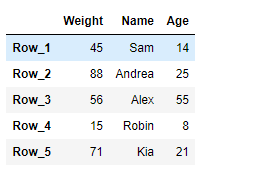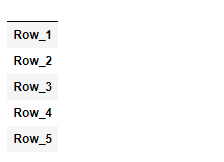GeeksforGeeks App
Open AppBrowser
Continue

# Python | Pandas DataFrame.empty

Pandas DataFrame is a two-dimensional size-mutable, potentially heterogeneous tabular data structure with labeled axes (rows and columns). Arithmetic operations align on both row and column labels.

It can be thought of as a dict-like container for Series objects. This is the primary data structure of the Pandas. Pandas DataFrame.empty attribute checks if the dataframe is empty or not. It returns True if the dataframe is empty else it returns False in Python.

In this article we will see How to Check if Pandas DataFrame is Empty.

## Pandas – Check if DataFrame is Empty

Syntax: DataFrame.empty

Parameter: None

Returns: Boolean Type

## Python3

 `# importing pandas as pd``import` `pandas as pd` `# Creating the DataFrame``df ``=` `pd.DataFrame({``'Weight'``:[``45``, ``88``, ``56``, ``15``, ``71``],``                   ``'Name'``:[``'Sam'``, ``'Andrea'``, ``'Alex'``, ``'Robin'``, ``'Kia'``],``                   ``'Age'``:[``14``, ``25``, ``55``, ``8``, ``21``]})` `# Create the index``index_ ``=` `[``'Row_1'``, ``'Row_2'``, ``'Row_3'``, ``'Row_4'``, ``'Row_5'``]` `# Set the index``df.index ``=` `index_` `# Print the DataFrame``print``(df)`

Output :## Use DataFrame.empty attribute

Example 1:

Here we will use DataFrame.empty attribute to check if the given dataframe is empty or not.

## Python3

 `# check if there is any element``# in the given dataframe or not``result ``=` `df.empty` `# Print the result``print``(result)`

Output :

` False`

Note: As we can see in the output, the DataFrame.empty attribute has returned False indicating that the given dataframe is not empty.

Example 2:

Now we will use DataFrame.empty attribute to check if the given dataframe is empty or not.

## Python3

 `# importing pandas as pd``import` `pandas as pd` `# Creating an empty DataFrame``df ``=` `pd.DataFrame(index ``=` `[``'Row_1'``, ``'Row_2'``,``                           ``'Row_3'``, ``'Row_4'``,``                           ``'Row_5'``])` `# Print the DataFrame``print``(df)` `# check if there is any element``# in the given dataframe or not``result ``=` `df.empty` `# Print the result``print``(result)`

Output :` True `

Note: As we can see in the output, the DataFrame.empty attribute has returned True indicating that the given dataframe is empty.

## Using dataframe.shape

Here we are using the dataframe’s shape that gives us the count of number of rows and number of columns.

## Python3

 `import` `pandas as pd``import` `numpy as np` `# Creating an empty DataFrame``df ``=` `pd.DataFrame({``'A'` `: [np.nan]})` `print``(df.dropna().shape[``0``] ``=``=` `0``)`

Output :

` True `

My Personal Notes arrow_drop_up